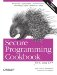# Working with Polar Coordinates

Problem

You want to represent and manipulate polar coordinates.

Solution

The complex template from the header provides functions for conversion to and from polar coordinates. Example 11-34 shows how you can use the complex template class to represent and manipulate polar coordinates.

Example 11-34. Using complex template class to represent polar coordinates

```#include
#include

using namespace std;

int main( ) {
double rho = 3.0; // magnitude
double theta = 3.141592 / 2; // angle
complex coord = polar(rho, theta);
cout << "rho = " << abs(coord) << ", theta = " << arg(coord) << endl;
coord += polar(4.0, 0.0);
cout << "rho = " << abs(coord) << ", theta = " << arg(coord) << endl;
}```

Example 11-34 produces the following output:

```rho = 3, theta = 1.5708
rho = 5, theta = 0.643501```

Discussion

There is a natural relationship between polar coordinates and complex numbers. Even though the two are somewhat interchangeable, it is generally not a good idea to use the same type to represent different concepts. Since using the complex template to represent polar coordinates is inelegant, I have provided a polar coordinate class that is more natural to use in Example 11-35.

Example 11-35. A polar coordinate class

```#include
#include

using namespace std;

template
struct BasicPolar
{
public:
typedef BasicPolar self;

// constructors
BasicPolar( ) : m( ) { }
BasicPolar(const self& x) : m(x.m) { }
BasicPolar(const T& rho, const T& theta) : m(polar(rho, theta)) { }

// assignment operations
self operator-( ) { return Polar(-m); }
self& operator+=(const self& x) { m += x.m; return *this; }
self& operator-=(const self& x) { m -= x.m; return *this; }
self& operator*=(const self& x) { m *= x.m; return *this; }
self& operator/=(const self& x) { m /= x.m; return *this; }
operator complex( ) const { return m; }

// public member functions
T rho( ) const { return abs(m); }
T theta( ) const { return arg(m); }

// binary operations
friend self operator+(self x, const self& y) { return x += y; }
friend self operator-(self x, const self& y) { return x -= y; }
friend self operator*(self x, const self& y) { return x *= y; }
friend self operator/(self x, const self& y) { return x /= y; }

// comparison operators
friend bool operator==(const self& x, const self& y) { return x.m == y.m; }
friend bool operator!=(const self& x, const self& y) { return x.m != y.m; }
private:
complex m;
};

typedef BasicPolar Polar;

int main( ) {
double rho = 3.0; // magnitude
double theta = 3.141592 / 2; // angle
Polar coord(rho, theta);
cout << "rho = " << coord.rho( ) << ", theta = " << coord.theta( ) << endl;
coord += Polar(4.0, 0.0);
cout << "rho = " << coord.rho( ) << ", theta = " << coord.theta( ) << endl;
system("pause");
}```

In Example 11-35, I have defined the Polar type as a typedef'd specialization of the BasicPolar template. This way you can have a convenient default but you can still specialize the BasicPolar template using another numerical type if you prefer. This technique is used in the standard library with the string class being a specialization of the basic_string template.Secure Programming Cookbook for C and C++: Recipes for Cryptography, Authentication, Input Validation & More
ISBN: 0596003943
EAN: 2147483647
Year: 2006
Pages: 241